Next: Steady Flow over a Up: Waves in Incompressible Fluids Previous: Gravity Waves in a

# Gravity Waves at an Interface

Consider a layer of fluid of density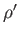, depth, and uniform horizontal velocity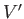, situated on top of a layer of another fluid of density, depth, and uniform horizontal velocity. Suppose that the fluids are bounded from above and below by rigid horizontal planes. Let these planes lie at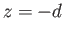and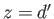, and let the unperturbed interface between the two fluids lie at. (See Figure 11.8.)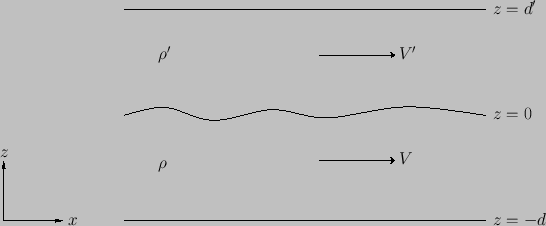Consider a gravity wave of angular frequency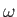, and wavenumber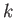, propagating through both fluids in the-direction. Let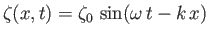(11.94)

be the small vertical displacement of the interface due to the wave. In the lower fluid, the perturbed velocity potential must be of the form (11.91), with the constantsand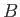chosen such thatand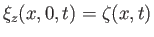. It follows thatand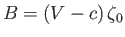, so that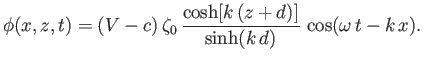(11.95)

In the upper fluid, the perturbed velocity potential must again be of the form (11.91), with the constantsandchosen such that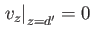and. It follows thatand, so that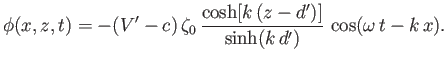(11.96)

Here,is the phase velocity of the wave. From Equations (11.85) and (11.92), the fluid pressure just below the interface is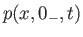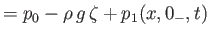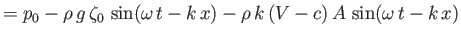(11.97)

Likewise, the fluid pressure just above the interface is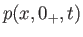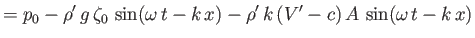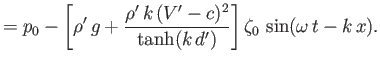(11.98)

In the absence of surface tension at the interface, these two pressure must equal one another: that is,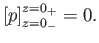(11.99)

Hence, we obtain the dispersion relation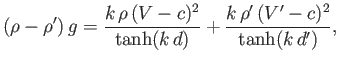(11.100)

which takes the form of a quadratic equation for the phase velocity,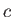, of the wave. We can see that:
1. If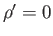and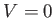then the dispersion relation reduces to (11.43) (with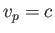).
2. If the two fluids are of infinite depth then the dispersion relation simplifies to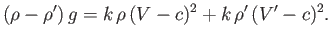(11.101)

3. In general, there are two values ofthat satisfy the quadratic equation (11.100). These are either both real, or form a complex conjugate pair.
4. The condition for stability is thatis real. The alternative is thatis complex, which implies thatis also complex, and, hence, that the perturbation grows or decays exponentially in time. Because the complex roots of a quadratic equation occur in complex conjugate pairs, one of the roots always corresponds to an exponentially growing mode. In other words, an instability.
5. If both fluids are at rest (i.e.,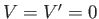), and of infinite depth, then the dispersion reduces to(11.102)

It follows that the configuration is only stable when: that is, when the heavier fluid is underneath.

As a particular example, suppose that the lower fluid is water, and the upper fluid is the atmosphere. Let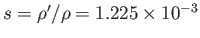be the specific density of air at s.t.p. (relative to water). Putting,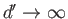, and making use of the fact thatis small, the dispersion relation (11.100) yields(11.103)

Comparing this with Equation (11.43), we can see that the presence of the atmosphere tends to slightly diminish the phase velocities of gravity waves propagating over the surface of a body of water.Next: Steady Flow over a Up: Waves in Incompressible Fluids Previous: Gravity Waves in a
Richard Fitzpatrick 2016-03-31